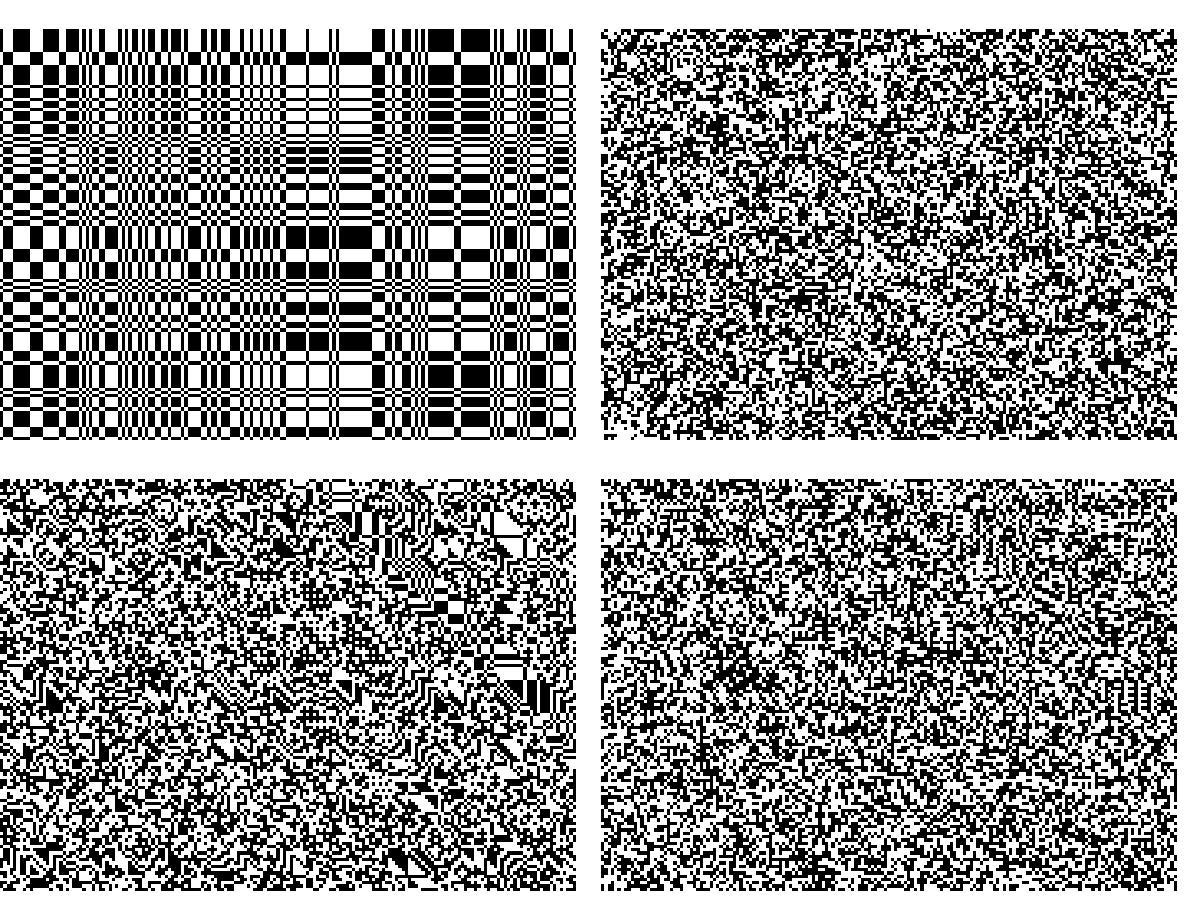# K-wise independent percolationA site percolation in an N x N box is an assignment of the value open or closed to each point (also called site) of the box. One typically talks about independent percolation in which each site is randomly determined to be open or closed with probability p, independently between sites, a sample with p=1/2 is shown on the top right part of the picture at the top of this page (open is displayed as black, closed as white). One is usually interested in the event that there is a path of open sites from the left side of the box to the right side of the box, this is called the event of percolation crossing and is also well defined for boxes in higher dimensions. It is well known that for independent percolation, for each dimension there is a critical probability p_c such that if p>p_c the probability of percolation crossing tends to 1 with N and if p<p_c the probability tends to 0.

One can also consider other distributions on the sites, for example consider an N x N box and attach to each horizontal and each vertical line a uniform sign (+1 or -1 with probability 1/2) independently, then the site at position (x,y) will be open iff the signs corresponding to x and y are the same. This leads to a picture such as the one on the top left part of the picture at the top of the page. As one clearly sees, this model differs significantly from the fully independent model, still, there is one sense in which the two are the same, if one considers any three sites in the new model then their distribution is just the uniform independent distribution (but there are choices of four sites which exhibit dependence). We then say that this model is 3-wise independent.

Similarly, at the bottom left part of the top picture we see another model on the N x N box, in which in addition to the signs attached to each horizontal and vertical lines we have a sign attached to each diagonal line, then a site is open if the product of the signs of the horizontal, vertical and diagonal lines passing through it is 1. This model is called trixor and was invented by Itai Benjamini. As one sees it too differs visually from the fully independent model, though less so than the previous model, and checking the definition one sees that it is 5-wise independent (that is, any 5 sites have the uniform independent distribution and there are choices of 6 sites which are dependent).

The picture on the bottom right part of the top picture is anti-trixor invented by Omer Angel. This picture does not seem visually different from the fully independent model (at the top right part), but amazingly, it is just a deterministic function of the trixor picture, more precisely, there is a fixed periodic picture of black and white such that the anti-trixor picture is obtained from the trixor picture by flipping all of the sites in which the fixed pattern is black (the particular sample on the bottom right was obtained from the sample on the bottom left in this way). Even more amazingly, it appears that global statistical properties of the anti-trixor picture (such as the crossing event) behave the same as the fully independent model and it is even conjectured that they have the same scaling limit as N tends to infinity (involving SLE(6))! For more on this see the nice presentation (Warning: large file) by Gábor Pete.

The restriction of k-wise independence on a model is a local one, requiring that every k sites are independent, the examples in the top picture show that models with this local restriction can behave very differently globally than the fully independent model. Still, these are examples for small values of k, do there exist models with high values of k which still behave very differently globally? Asking a concrete question, fix p=0.01 meaning that approximately 1% of the sites are open (this is well below p_c for the square), is it true that for any k, any k-wise independent percolation with this p has small probability of crossing (when N is large)?
In joint work with Ori Gurel-Gurevich and Itai Benjamini we answer this question in the negative (among other results on k-wise independent distributions), we construct for any k and any 0<p<1 (if N is large enough) an example of a k-wise independent percolation with this probability p that has probability 1 of crossing, and another example which has probability 0 of crossing. We furthermore roughly identify how high can k be as a function of N for this phenomena to exist, we find (very roughly) that when k is less than log(N) we can have such distributions and when k is more than log(N) such distributions do not exist. In the picture below, on the left, we see a sample from such a distribution, for ease of simulation the picture is for a bond percolation, that is when every edge between two adjacent sites is open or closed, with parameters k=2 and p=1/3, for comparison we see on the right a sample from the fully independent model. Observe that indeed we have a crossing from left to right (highlighted with the box) in the 2-wise independent model. The examples we construct can also be extended to higher dimensions, and to the entire R^d. Thus we can have for any d,k and p, a translation invariant and ergodic (site or bond) percolation on R^d which has probability 1 of an infinite open cluster somewhere, or another distribution with probability 0 for an infinite open cluster.